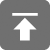# 工学1号馆

home

## java核心系列6-继承

Wu Yudong    June 10, 2015     Java   455

### 继承的实现

class 子类名 extends 父类名{
//新增属性、方法，或改写父类原有方法
}


Java只支持单一继承，不允许多重继承，即：

//B类继承A类
class A{				//父类
int x=10;
public void setX(int x){
this.x=x;
}
public int getX(){
return x;
}
public String toString(){
String information="";
information=information+"x= "+x+"\n";
return information;
}
}
class B extends A{			//子类继承父类
int y=100;			//子类新增属性
public void setY(int y){	//子类新增方法
this.y=y;
}
public int getY(){		//子类新增方法
return y;
}
public String toString(){	//子类改写父类方法
String information="";
information=information+"x= "+x+"\n";
information=information+"y= "+y+"\n";
return information;
}
}
public class InheritTest {
public static void main(String args[]) {
A a=new A();
B b=new B();
System.out.println(a.toString());
System.out.println(b.toString());
}
}


### 重用

public class Employee{
private String name;
private int age;
private double salary;

Employee (String name, int age,double salary){
this.name=name;
this.age=age;
this.salary=salary;
}
Employee (){
this(null,0,0);
}
public  double getSalary(){
return salary;
}
public void setSalary(double salary){
this. salary =  salary;
}
public double getIncome (){
return salary;
}
}


public class Manager{
private Employee employee;
private double bonus;

Manager (String name, int age,double salary,double bonus){
employee =new Employee(name,age,salary);
this.bonus=bonus;
}
Manager (){
this(null,0,0,0);
}
……
}


1.组合是在类中，定义要复用的类的对象，作为属性。

2.通过这个属性达到复用目的。类似于一种代码组合。

3.它们之间的关系是has-a关系

public class Manager extends Employee{
private double bonus;

Manager (String name, int age,double salary,double bonus){
super(name,age,salary);
this.bonus=bonus;
}
Manager (){
this(null,0,0,0);
}
……
}


### 变量隐藏与方法覆盖

Java允许在子类定义与父类同名(类型可以不同)的变量，在子类中直接使用这一变量名时，访问的是子类定义的变量，而父类同名的变量则被隐藏起来，这称为变量的隐藏

Java还允许在子类中对父类原有的方法进行重写，以实现新的功能。

• 重写方法和被重写方法必须具有相同的方法名；
• 重写方法和被重写方法必须具有相同的参数列表；
• 重写方法的返回值类型必须和被重写方法的返回值类型相同或者是其子类；
• 重写方法的不能缩小被重写方法的访问权限。
• 子类方法不能抛出比父类方法更多的异常。

public class Point {
public  int x, y;
public String toString(){…}
}
public class Circle extends Point {
public int x, y ,radius;  // 变量隐藏
public String toString(){…}  //方法覆盖
public String toString(int x, int y, int radius){…}  //方法重载
}


//B类继承A类
class A{					//父类
int x=10;                                                             //被隐藏的变量
public void setX(int x){
this.x=x;
}
public int getX(){
return x;
}
public String toString(){                                  //被覆盖的方法
String information="";
information=information+"x= "+x+"\n";
return information;
}
}
//父类代码与前面相同，这里省略不写
class B extends A{				//子类继承父类
double x=-12.345			//变量隐藏
int y=100;				//子类新增属性
public void setY(int y){			//子类新增方法
this.y=y;
}
public int getY(){			//子类新增方法
return y;
}
public String toString(){			//子类改写父类方法,即方法覆盖
String information="";
information=information+"x= "+x+"\n";
information=information+"y= "+y+"\n";
return information;
}
}
public class InheritTest {
public static void main(String args[]) {
A a=new A();
B b=new B();
System.out.println(a.toString());
System.out.println(b.toString());
}
}


x= 10

x= -12.345

y= 100

### super关键字

Super 可用来引用父类的成分，它有两种主要用法：

super.变量

super([…])；//与this用法类似，应放在构造方法的第一行位置上。

class A{
public A(){
System.out.println("调用了A类的构造方法");
}
}
class B extends A{
public B(){
System.out.println("调用了B类的构造方法");
}
}
public class C extends B {
public C(){
System.out.println("调用了C类的构造方法");
}
public static void main(String args[]) {
C c=new C();
}
}


//private权限类
class Person {
private String name="";		//私有访问权限
private int age=0;
public Person(String name, int age){
this.name=name;
this.age=age;
}
public String getName(){
return name;
}
public int getAge(){
return age;
}
}
class Student extends Person{
public Student(String name, int age){
super(name, age);
}
public String toString(){
String str="";
str=str+"姓名："+name;
str=str+", 年龄："+age;
return str;
}
}
public class PrivateTest {
public static void main(String args[]){
Student wang=new Student("王小丽", 20);
System.out.println(wang.toString());
}
}